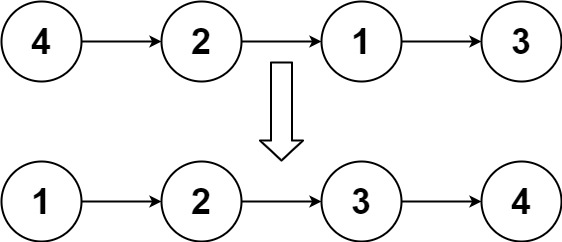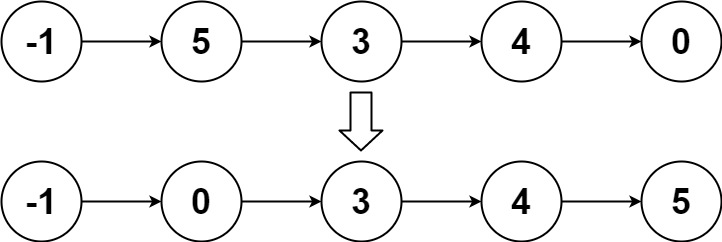## 148. Sort List

Given the `head` of a linked list, return the list after sorting it in ascending order.

Follow up: Can you sort the linked list in `O(n logn)` time and `O(1)` memory (i.e. constant space)?

Example 1:```Input: head = [4,2,1,3]
Output: [1,2,3,4]
```

Example 2:```Input: head = [-1,5,3,4,0]
Output: [-1,0,3,4,5]
```

Example 3:

```Input: head = []
Output: []
```

Constraints:

• The number of nodes in the list is in the range `[0, 5 * 104]`.
• `-105 <= Node.val <= 105`

## Rust Solution

``````struct Solution;
use rustgym_util::*;

impl Solution {
let mut v: Vec<i32> = vec![];
while let Some(node) = cur {
v.push(node.val);
cur = node.next;
}
v.sort_unstable();
let mut prev = None;
while let Some(last) = v.pop() {
}
prev
}
}

#[test]
fn test() {
let head = list![4, 2, 1, 3];
let res = list![1, 2, 3, 4];